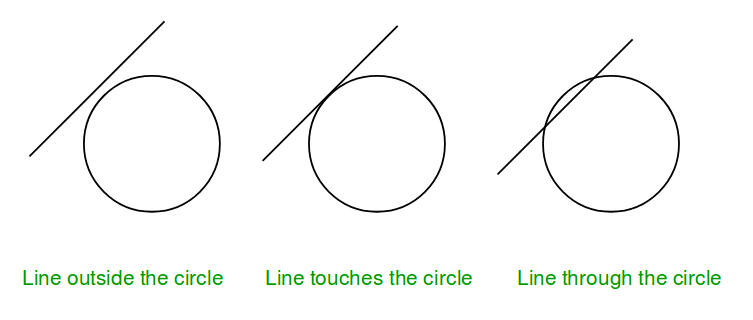Open In App
Related Articles

# Check if a line touches or intersects a circle

Given coordinate of the center and radius > 1 of a circle and the equation of a line. The task is to check if the given line collides with the circle or not. There are three possibilities :

1. Line intersects the circle.
2. Line touches the circle.
3. Line is outside the circleNote: General equation of a line is a*x + b*y + c = 0, so only constant a, b, c are given in the input.

Examples :

Input : radius = 5, center = (0, 0),         a = 1, b = -1, c = 0.Output : IntersectInput :  radius = 5, center = (0, 0),          a = 5, b = 0, c = 0.Output : IntersectInput : radius = 5, center = (0, 0),         a = 1, b = 1, c = -16.Output : Outside

The idea is to compare the perpendicular distance between center of circle and line with the radius of the circle.
Algorithm:
1. Find the perpendicular (say p) between center of circle and given line.
2. Compare this distance p with radius r.
……a) If p > r, then line lie outside the circle.
……b) If p = r, then line touches the circle.
……c) If p < r, then line intersect the circle.
How to find the perpendicular distance?
Distance of a line from a point can be computed using below formula:## C++

 // CPP program to check if a line touches or// intersects or outside a circle.#include using namespace std; void checkCollision(int a, int b, int c,                  int x, int y, int radius){    // Finding the distance of line from center.    int dist = (abs(a * x + b * y + c)) /                     sqrt(a * a + b * b);     // Checking if the distance is less than,    // greater than or equal to radius.    if (radius == dist)        cout << "Touch" << endl;    else if (radius > dist)        cout << "Intersect" << endl;    else        cout << "Outside" << endl;} // Driven Programint main(){    int radius = 5;    int x = 0, y = 0;    int a = 3, b = 4, c = 25;    checkCollision(a, b, c, x, y, radius);    return 0;}

## Java

 // Java program to check if a line touches or// intersects or outside a circle. import java.io.*; class GFG {         static void checkCollision(int a, int b, int c,                               int x, int y, int radius)    {        // Finding the distance of line from center.        double dist = (Math.abs(a * x + b * y + c)) /                        Math.sqrt(a * a + b * b);             // Checking if the distance is less than,        // greater than or equal to radius.        if (radius == dist)            System.out.println ( "Touch" );        else if (radius > dist)            System.out.println( "Intersect") ;        else            System.out.println( "Outside") ;    }         // Driven Program    public static void main (String[] args)    {        int radius = 5;        int x = 0, y = 0;        int a = 3, b = 4, c = 25;        checkCollision(a, b, c, x, y, radius);         }} // This article is contributed by vt_m.

## Python3

 # python program to check if a line# touches or  intersects or outside# a circle. import math def checkCollision(a, b, c, x, y, radius):         # Finding the distance of line    # from center.    dist = ((abs(a * x + b * y + c)) /            math.sqrt(a * a + b * b))     # Checking if the distance is less    # than, greater than or equal to radius.    if (radius == dist):        print("Touch")    elif (radius > dist):        print("Intersect")    else:        print("Outside") # Driven Programradius = 5x = 0y = 0a = 3b = 4c = 25checkCollision(a, b, c, x, y, radius) # This code is contributed by Sam007

## C#

 // C# program to check if a line touches or// intersects or outside a circle.using System; class GFG {         static void checkCollision(int a, int b, int c,                            int x, int y, int radius)    {        // Finding the distance of line from center.        double dist = (Math.Abs(a * x + b * y + c)) /                        Math.Sqrt(a * a + b * b);             // Checking if the distance is less than,        // greater than or equal to radius.        if (radius == dist)            Console.WriteLine ("Touch");        else if (radius > dist)            Console.WriteLine("Intersect");        else            Console.WriteLine("Outside");    }         // Driven Program    public static void Main ()    {        int radius = 5;        int x = 0, y = 0;        int a = 3, b = 4, c = 25;                 checkCollision(a, b, c, x, y, radius);         }} // This article is contributed by vt_m.

## Javascript

 

## PHP

  $dist) echo "Intersect"; else echo "Outside" ;} // Driver Code$radius = 5;$x = 0;$y = 0;$a = 3;$b = 4;$c = 25;checkCollision($a, $b, $c, $x, $y, \$radius); // This code is contributed by Sam007?>

Output

Touch


Time Complexity : O(log(a*a + b*b)) as it is using inbuilt sqrt function
Auxiliary Space : O(1)

This article is contributed by Anuj Chauhan. If you like GeeksforGeeks and would like to contribute, you can also write an article using write.geeksforgeeks.org or mail your article to review-team@geeksforgeeks.org. See your article appearing on the GeeksforGeeks main page and help other Geeks.